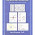## Wednesday, 15 June, 2011

### ATOMIC ORBITAL

An atomic orbital is a mathematical function that describes the wave-like behavior of either one electron or a pair of electrons in an atom. This function can be used to calculate the probability of finding any electron of an atom in any specific region around the atom's nucleus. The term may also refer to the physical region defined by the function where the electron is likely to be.
Within a physical context atomic orbitals are the basic building blocks of the electron cloud model (alternatively referred to as the wave mechanics model or atomic orbital model), a modern framework for describing the placement of electrons in an atom. In this model, the atom consists of a nucleus surrounded by orbiting electrons. These electrons exist in atomic orbitals, which are a set of quantum states of the negatively charged electrons trapped in the electrical field generated by the positively charged nucleus. The electron cloud model can only be described by quantum mechanics, in which the electrons are most accurately described as standing waves surrounding the nucleus.

Atomic orbitals are typically described as “hydrogen-like” (meaning one-electron) wave functions over space, categorized by n, l, and m quantum numbers, which correspond to the electrons' energy, angular momentum, and an angular momentum direction, respectively. Each orbital is defined by a different set of quantum numbers and contains a maximum of two electrons. The simple names s orbital, p orbital, d orbital and f orbital refer to orbitals with angular momentum quantum number l = 0, 1, 2 and 3 respectively. These names indicate the orbital shape and are used to describe the electron configurations. They are derived from the characteristics of their spectroscopic lines: sharp, principal, diffuse, and fundamental, the rest being named in alphabetical order (omitting j).

The wavefunction for the electron cloud of a complex atom may be seen as being built up (in approximation) in an electron configuration that is a sum of simpler hydrogen-like atomic orbitals. In such a configuration, pairs of electrons are arranged in simple repeating patterns of increasing odd numbers (1,3,5,7..), each of which represents a set of electron pairs with a given energy and angular momentum. The repeating periodicity of the blocks of 2, 6, 10, and 14 elements in the periodic table arises naturally from the total number of electrons which occupy a complete set of s, p, d and f atomic orbitals, respectively.

#### 1 comment:

1.Very good illustration